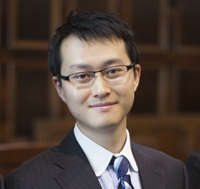The Student Puzzle Corner contains one or two problems in statistics or probability. Sometimes, solving the problems may require a literature search.
Current student members of the IMS are invited to submit solutions electronically (to bulletin@imstat.org with subject “Student Puzzle Corner”). Deadline August 15, 2014.
The names and affiliations of (up to) the first 10 student members to submit correct solutions, and the answer(s) to the problem(s), will be published in the next issue of the Bulletin. The Editor’s decision is final.

## Student Puzzle Corner 5

The problem in the last issue was on statistics. This time we pose a problem on probability.

Suppose couples in a certain country have a Poisson number of children with mean λ. Little Dennis is a son of the Mitchells. For what values of λ would you bet that Dennis has an equal number of brothers and sisters? Assume, as is usual, that childbirths are independent and that each birth results in a boy or a girl with probability ½ each.

It is a little difficult to get reliable data on number of children per couple in various countries. It is easier to get some data on the average number of children per woman. For example, in the US, it seems to be about 1.8 among whites; about 0.8 in Singapore; about 1.2 in the Czech Republic; 1.4 in Japan, Germany and Greece; 1.5 in Switzerland; 1.6 in Canada and Russia; 1.8 in Brazil, Norway and Australia; in the UK it’s about 1.9; 2.0 in France; 2.5 in India; 2.6 in Israel; 2.9 in Egypt; 3.3 in Jordan; 4.4 in Madagascar; 5.0 in Tanzania; 6.0 in Uganda; 7.0 in Niger. The worldwide average is about 2.5.## Last issue’s Student Puzzle

Suppose a parameter μ was measured at two different laboratories, of which one is more renowned and reliable than the other. Formally, X ~ N(μ, 1), Y ~ N(μ, σ2), where X, Y are independent, and $σ^2$≥1. Find, explicitly, a 95% confidence interval of finite length for $σ^2$. It seems a little odd at first that one can estimate the variance of the second laboratory with only one observation from the second laboratory. In some sense, a more basic question is how will you estimate μ in such a case, or what are the maximum likelihood estimates of μ, $σ^2$, but they are not being asked here.

Anirban DasGupta, IMS Bulletin Editor, explains:

Peng Ding (pictured below) of the Statistics Department at Harvard University sent a correct—and nicely written—solution to the problem asked. We encourage more of our student members to send solutions!Suppose $X \sim N(\mu ,1), Y \sim N(\mu , \sigma ^2)$, where $X, Y$ are independent, the parameters $\mu, \sigma ^2$ are both unknown, but it is known that $\sigma ^2 \geq 1$. Such a problem might arise if an unknown parameter $\mu$ was measured at two laboratories, of which one is more reliable and established than the other one. The problem asked in the puzzle of the last issue was to construct a $95\%$ confidence interval for $\sigma ^2$ of finite length. There are infinitely many $95\%$ confidence intervals for $\sigma ^2$ with such data, but some have infinite length, i.e., they really are one sided intervals. But there are also confidence intervals of finite length.
\\
To construct a confidence interval for $\sigma ^2$, notice that $Y-X \sim N(0, 1+\sigma ^2)$; that $Y-X$ has a distribution free of $\mu$, i.e., it is a {\it partial ancillary}, enables the construction of confidence intervals for $\sigma ^2$ although there is only one observation from the second laboratory.
\\
We may as well solve the problem for a general confidence level $1-\alpha , 0 < \alpha < 1$. Denote the $\alpha /2$th quantile of a $\chi _1^2$ distribution by $a$ and the $(1-\alpha /2)$th quantile of $\chi _1^2$ by $b$. Thus, $P(a \leq \chi _1^2 \leq b) = 1-\alpha$. Since $\frac{(Y-X)^2}{1+\sigma ^2} \sim \chi _1^2$, this leads to $1-\alpha = P(a \leq \frac{(Y-X)^2}{1+\sigma ^2} \leq b)$ $= P(\frac{(Y-X)^2}{b} -1 \leq \sigma ^2 \leq \frac{(Y-X)^2}{a} -1).$ Since we know that $\sigma ^2 \geq 1$, this means that the interval $[\max\{\frac{(Y-X)^2}{b} -1, 1\}, \frac{(Y-X)^2}{a} -1]$ is an $100(1-\alpha )\%$ confidence interval for $\sigma ^2$, the interval being empty if $(Y-X)^2 <2a$. It would be an embarrassment to report an empty set as (say) a $95\%$ confidence interval if it were to happen. Procedures based on sample space calculations can, at times, give seemingly silly answers. What the answer is trying to tell you is that data contradict your model, i.e., there is no $\sigma ^2 \geq 1$ consistent with the data obtained. In contrast, Bayesian confidence intervals will never be empty, but will require writing down a prior. Much has been written on these foundational issues. We can calculate the probability that our confidence interval will be empty. It equals $P((Y-X)^2 < 2a) = P(\chi _1^2 < \frac{2a}{1+\sigma ^2})$ $= 2\Phi (\sqrt{\frac{2a}{1+\sigma ^2}}) -1 = \frac{2\sqrt{a}}{\sigma \sqrt{\pi }} + O(\sigma ^{-3}).$ For example, if $\alpha = .05$ and $\sigma ^2 = 2$, then the probability of reporting an empty confidence interval is about $.015$. The interval above is equal tailed; one may also find intervals that are not equal tailed. They may have certain advantages as regards the expected length. \\ It was mentioned in the puzzle that a more basic problem in this case is estimation of $\mu$. How should one combine the reports of the two laboratories? It may be shown that a unique maximum likelihood estimate for $\mu$ exists for all $X, Y$. However, this estimate is nonlinear. Of the two observations $X, Y, X$ is more reliable. The MLE takes the average of $X$ and $Y$ and shrinks it towards $X$. The amount of shrinkage depends on $(Y-X)^2$, i.e., how similar are the two lab results. The maximum likelihood estimate of $\sigma ^2$ will equal the boundary value $\sigma ^2 = 1$ with a positive probability under all $\sigma ^2$; once again, one can write it down exactly.